## Pairwise comparison

The residue by residue scores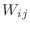can be used directly in the sequence alignment algorithm of Needleman & Wunsch [Needleman & Wunsch, 1970] to obtain the comparison of two protein sequences or structures. The only difference between the two types of comparison is in the type of the comparison matrix. In the case of sequence, the amino acid substitution matrix is used. In the case of 3D structure, the Euclidean distance (or some function of it) between two equivalent atoms in the current optimal superposition is used [Šali & Blundell, 1990].

The problem of the optimal alignment of two sequences as addressed by the algorithm of Needleman & Wunsch is as follows. We are given two sequences of elements and an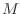times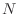score matrix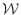whereandare the numbers of elements in the first and second sequence. The scoring matrix is composed of scoresdescribing differences between elements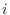and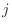from the first and second sequence respectively. The goal is to obtain an optimal set of equivalences that match elements of the first sequence to the elements of the second sequence. The equivalence assignments are subject to the following “progression rule”: for elementsand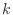from the first sequence and elementsand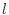from the second sequence, if elementis equivalenced to element, if elementis equivalenced to elementand ifis greater than,must also be greater than. The optimal set of equivalences is the one with the smallest alignment score. The alignment score is a sum of scores corresponding to matched elements, also increased for occurrences of non-equivalenced elements (ie gaps). For a detailed discussion of this and related problems see [Sankoff & Kruskal, 1983].

We summarize the dynamic programming formulae used by MODELLER to obtain the optimal alignment since they differ slightly from those already published [Sellers, 1974,Gotoh, 1982]. The recursive dynamic programming formulae that give a matrix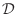are: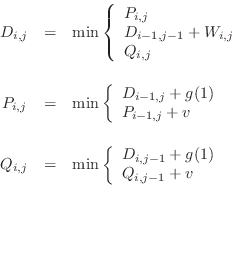(A.1)

where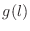is a linear gap penalty function: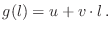(A.2)

Note that only a vector is needed for the storage ofand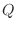. The uppermost formula in Eq. A.1 is calculated for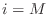and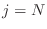. Variableis a gap length and parameters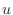and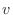are gap-penalty constants.

The arrays,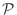and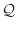are initialized as follows: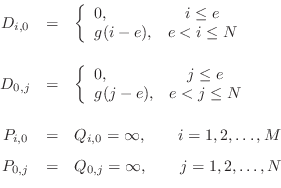(A.3)

where parameter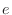is the maximal number of elements at sequence termini which are not penalized with a gap-penalty if not equivalenced. A segment at the terminus of lengthis termed an “overhang”. Note a difference from [Gotoh, 1982] in the initialization of theandarrays. Also note that only vectors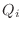and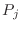need to be stored in computer, not the whole arrays.

The minimal score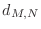is obtained from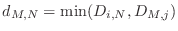(A.4)

where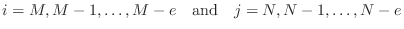to allow for the overhangs. The equivalence assignments are obtained by backtracking in matrix. Backtracking starts from the element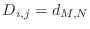.

Automatic builds 2017-07-19